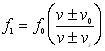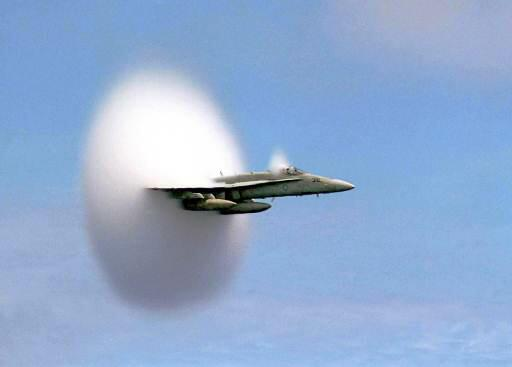#### The Doppler Effect and Sonic Booms

The animation below shows a simple representation of a stationary Sound Source

Sound radiates at a constant speed in all directions (concentrically) for a source.When you hear and ambulance approaching, its siren's pitch (frequency) changes as it comes near you and then speeds away from your standing point.

• The siren is the source of sound.
• The sound sounrce in this case is not stationary but it is in motion.
• The pitch of a moving sound source changes with respect to a stationary listener.

This effect was first explained in 1842 by Christian Doppler.

The Doppler Effect is the shift in frequency and wavelength of waves of a moving sound source with respect to the medium.

The Doppler effect can also be applied to a receiver (a listener) moving with respect to the medium, or even a medium that is in motion with respect to a sound source or receiver.

The frequency perceived by the listener (f1) is related to the actual frequency of the source (f0) and the relative speeds of the source (vs), observer (vo), and the speed (v) of waves in the medium as described mathematically by the following equation:Note: if the source and the observer are moving towards each other, then their relative speeds are negative with respect to each other and consequently,  the frequency (f1)  is higher than the actual frequency (f0). If the source and observer are moving away from each other the  frequency perceived by the listener (f1) is lower than the actual frequency of the source (f0).

The Doppler effect applies to all types of waves including light and other electromagnetic waves.

The following animantions show what happens to tye concentric wves  when the  source of sound is not sationary :

Case 1 - vsource < vsoundCase 2 - vsource = vsound#### Sonic Booms

Case 3 - vsource >  vsound

Here is the case where the speed of the source is greater than the speed of soundWhen an object travels fater than the speed of sound in air, a shock wave is created.

This is the case where a supersonic jet breaks the barrier of the speed of sound.

The image below is one of an F-18 at the exact instant it goes supersonic.